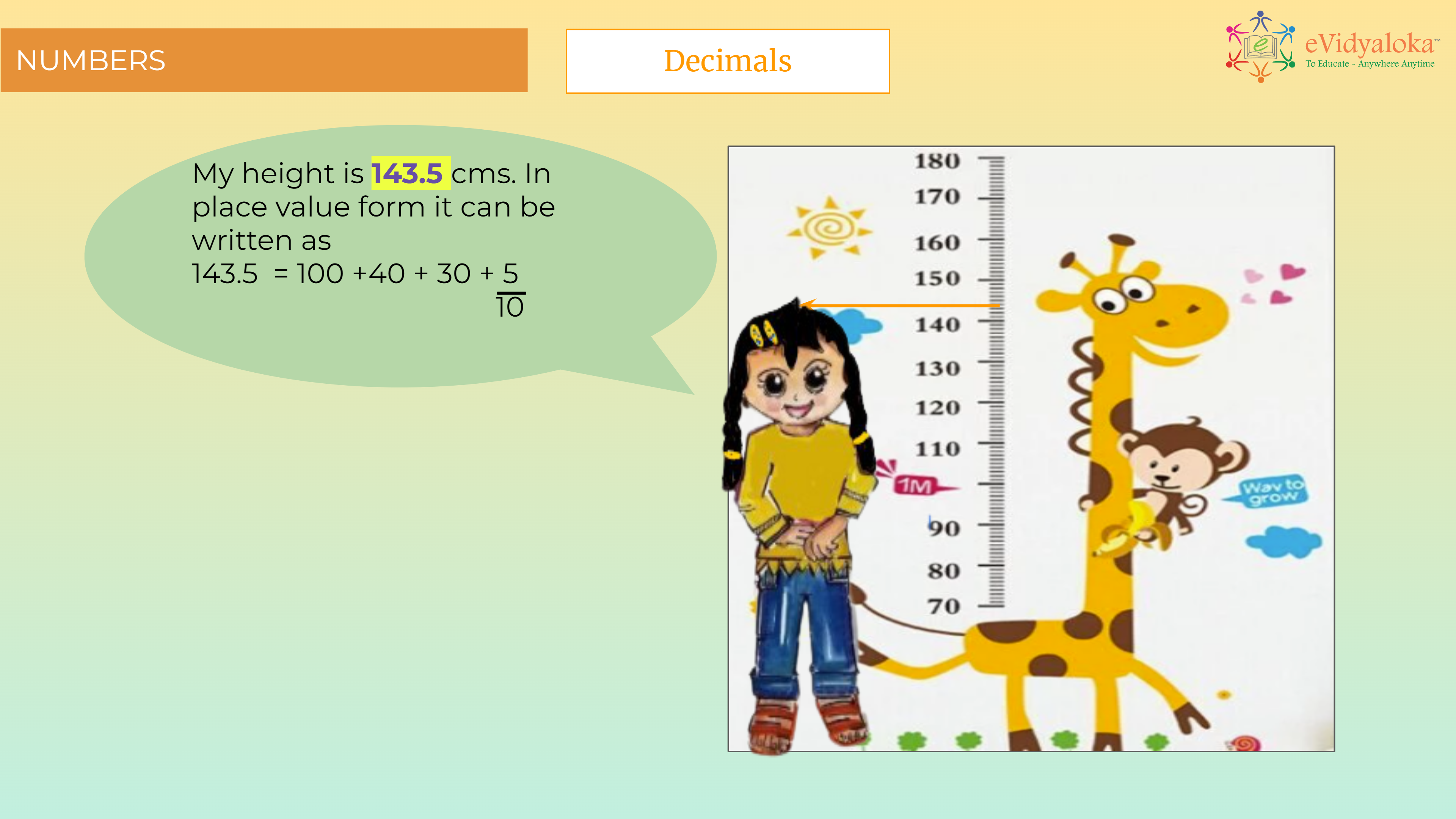# Fractions and Decimals

``````

Dear Volunteer Teacher,

Kindly teach the bridge concept of Fractions and Decimals prior to teaching the related textbook chapters.
Thank you.

## Course Content

• Introduction of Decimals
• Converting fractions into decimals
• Subtraction using decimals
• Multiplication using decimals
• Division using decimals
• Conversion of unit of length, mass and volume

## Learning Outcomes

Learning outcomes has been listed below which will serve as your guide for what students need to learn.

SWBAT:

• identify decimals and their place value.
• Convert decimals into fractions and perform operations on decimals.
• solve problems with units of measures (length, mass and volume).

## PPT Deck

To make the lesson come alive, please find below PPT deck